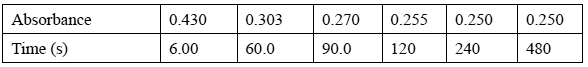Spectroscopy exercises (2007 VCE) When the substance CH3CHO (substance X) is dissolved in water it reacts to form an equilibrium mixture with CH3CH(OH)2 (substance Y) according to the equation X(aq) + H2O(l) => Y(aq) The concentration of X can be determined using UV-visible spectroscopy. X absorbs strongly at 290 nm Y shows no absorption at this wavelength. In a particular experimental arrangement at 25°C, the relationship between absorbance at 290 nm and concentration of X is given by Absorbance = 4.15 x [X] In the experiment, 0.110 mol of X is dissolved rapidly in 1.00 L of water at 25°C. The absorbance of the solution changes as some of the X is converted to Y. The table below shows the change in absorbance over time (measured in seconds).Calculate the concentration of X, in M, when the reaction reached equilibrium. Solution Calculate the absorbance at the instant that X was dissolved in the water, before any reaction occurred Solution Calculate the percentage of the original 0.110 mol of X that has been converted into Y at equilibrium. Solution The average rate of a reaction can be determined by calculating the change in concentration of a reactant per second. Calculate the average rate, in M/s, at which the concentration of X changed during the first 6.00 s of the reaction. Solution